# How to Calculate and Solve for r.p.m of Driver Pulley | Pulley Size Calculations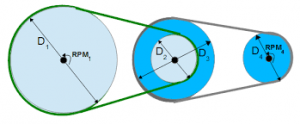The image above represents r.p.m of driver pulley.

To compute for r.p.m of driver pulley, three essential parameters are needed and these parameters are Diameter of Driven Pulley (Db), r.p.m. of Driven Pulley (rb) and Diameter of Driver Pulley (Db).

The formula for calculating r.p.m of driver pulley:

ra = (Db x rb/Da

Where:

ra = r.p.m. of Driver Pulley
Db = Diameter of Driven Pulley
rb = r.p.m. of Driven Pulley
Da = Diameter of Driver Pulley

Let’s solve an example;
Find the r.p.m of driver pulley when the diameter of driven pulley is 21, the r.p.m of driven pulley is 18 and the diameter of driver pulley is 14.

This implies that;

Db = Diameter of Driven Pulley = 21
rb = r.p.m. of Driven Pulley = 18
Da = Diameter of Driver Pulley = 14

ra = (Db x rb/Da
ra = (21 x 18)/14
ra = 378/14
ra = 27

Therefore, the r.p.m of driver pulley is 27 Hz.

Nickzom Calculator – The Calculator Encyclopedia is capable of calculating the r.p.m of driver pulley.

To get the answer and workings of the r.p.m of driver pulley using the Nickzom Calculator – The Calculator Encyclopedia. First, you need to obtain the app.

You can get this app via any of these means:

To get access to the professional version via web, you need to register and subscribe for NGN 2,000 per annum to have utter access to all functionalities.
You can also try the demo version via https://www.nickzom.org/calculator

Apple (Paid) – https://itunes.apple.com/us/app/nickzom-calculator/id1331162702?mt=8
Once, you have obtained the calculator encyclopedia app, proceed to the Calculator Map, then click on Materials and Metallurgical under Engineering.Now, Click on Ball Mill Sizing under Materials and MetallurgicalNow, Click on Pulley Size under Ball Mill Sizing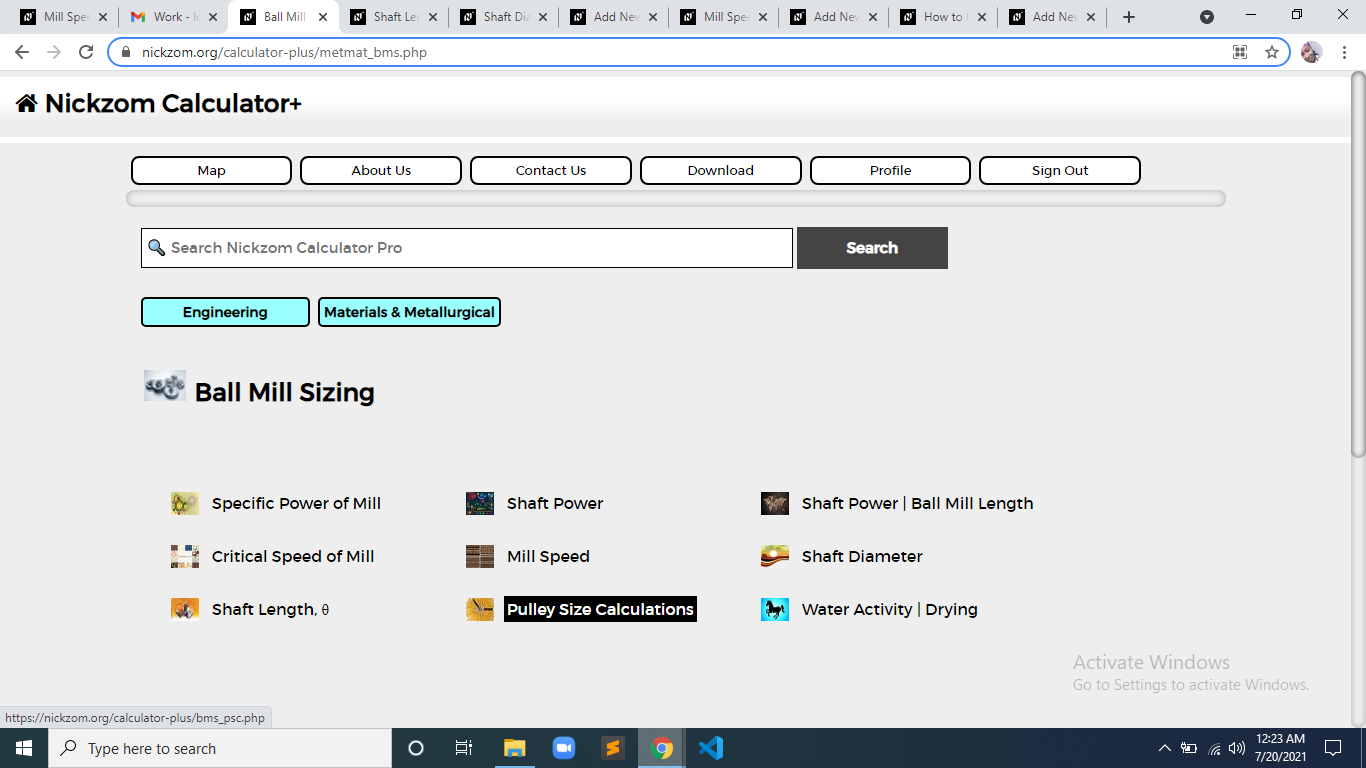Now, Click on r.p.m of Driver Pulley under Pulley Size Calculations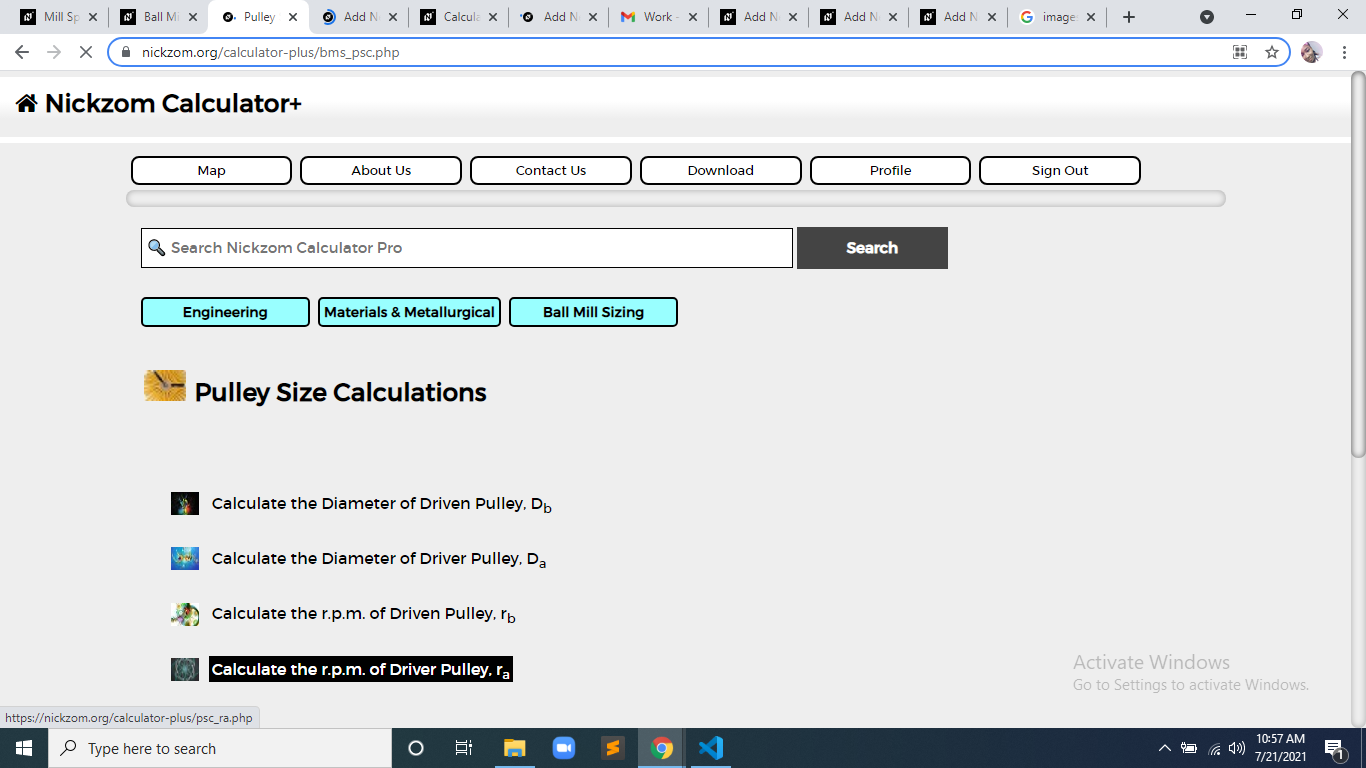The screenshot below displays the page or activity to enter your values, to get the answer for the r.p.m of driver pulley according to the respective parameters which is the Diameter of Driven Pulley (Db), r.p.m. of Driven Pulley (rb) and Diameter of Driver Pulley (Db).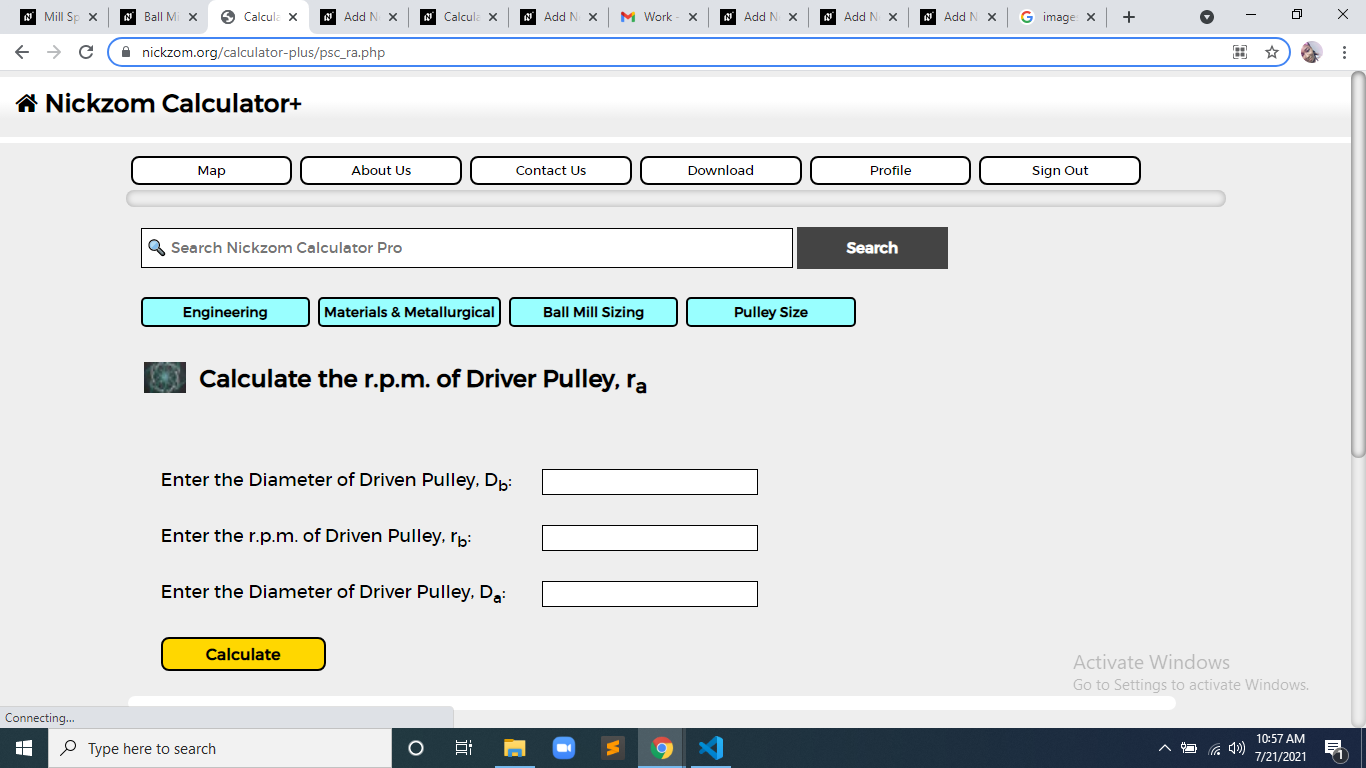Now, enter the value appropriately and accordingly for the parameter as required by the Diameter of Driven Pulley (Db) is 21, r.p.m. of Driven Pulley (rb) is 18 and Diameter of Driver Pulley (Db) is 14.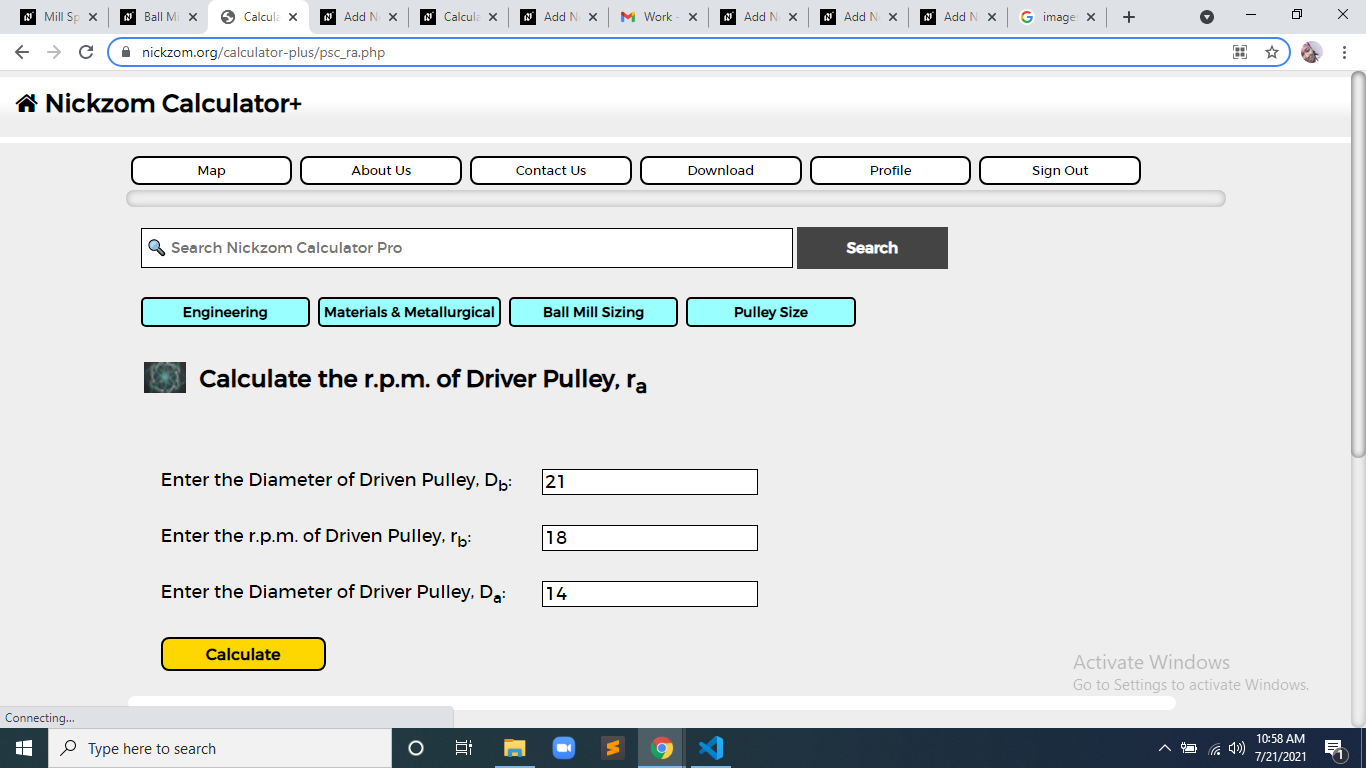Finally, Click on Calculate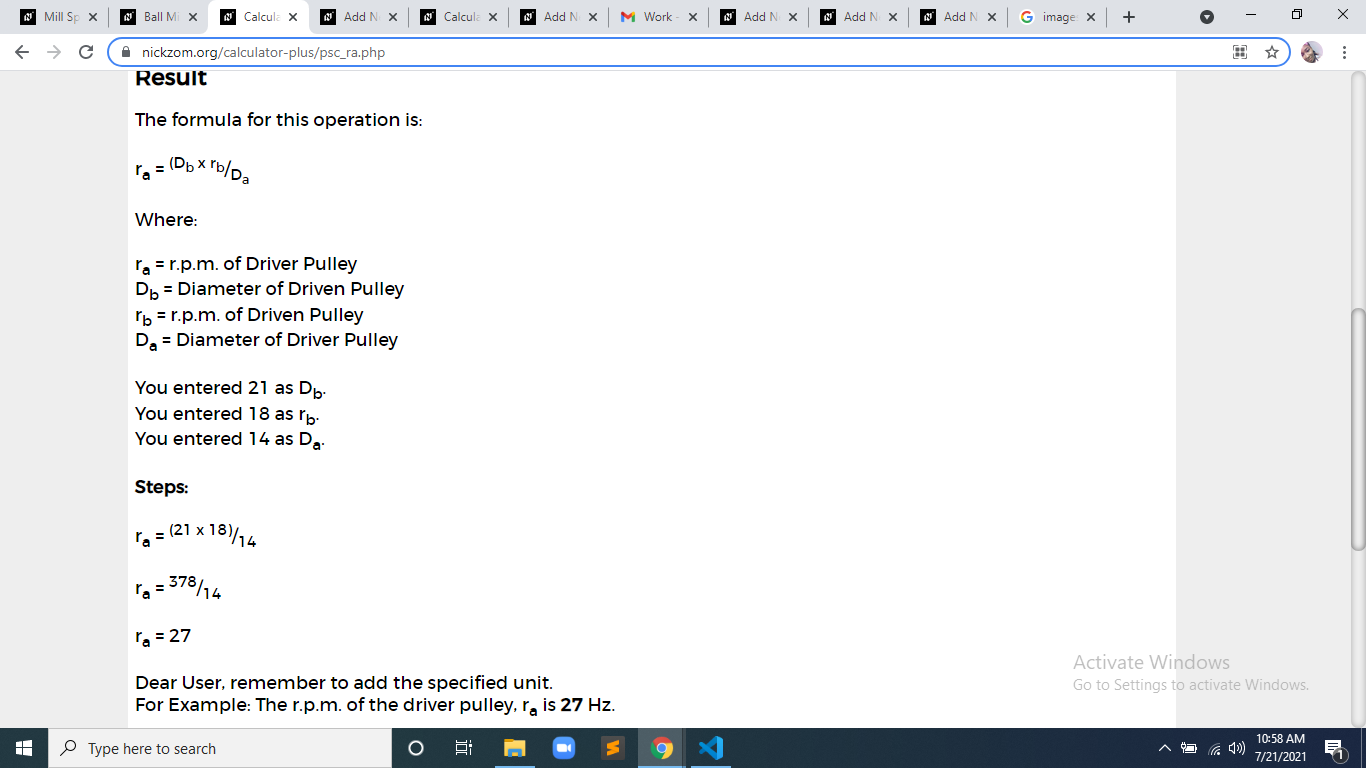As you can see from the screenshot above, Nickzom Calculator– The Calculator Encyclopedia solves for the r.p.m of driver pulley and presents the formula, workings and steps too.